#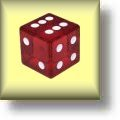Probability Worksheets

## Dynamically Created Probability Worksheets

These dynamically created Probability Worksheets are great for learning and practicing the concept of probability. These Probability Worksheets are ideal for 4th Grade, 5th Grade, 6th Grade, and 7th Grade students.

## Quick Link for All Probability Worksheets

Click the image to be taken to that Probability Worksheet.

##### Probability WorksheetsDefinitions of Probability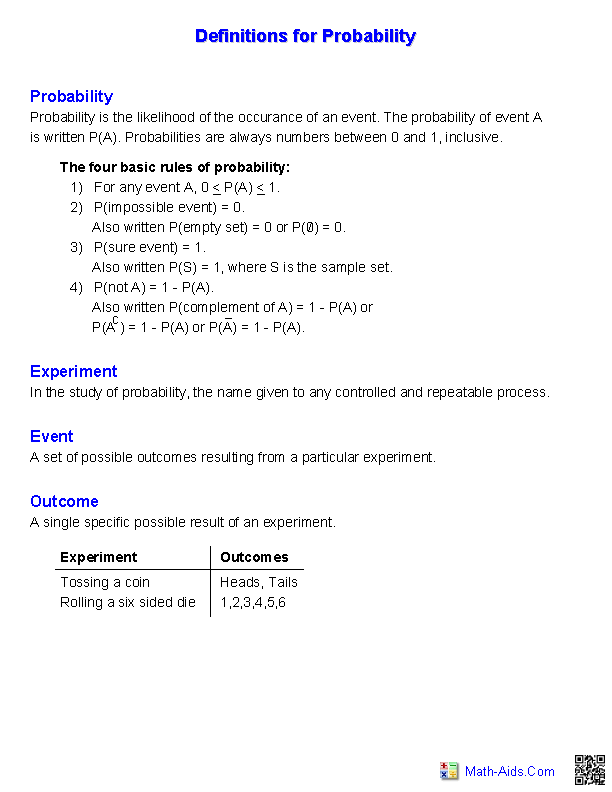##### Probability WorksheetsOn Numbers##### Probability WorksheetsWith a Single Die##### Probability WorksheetsWith a Pair of Dice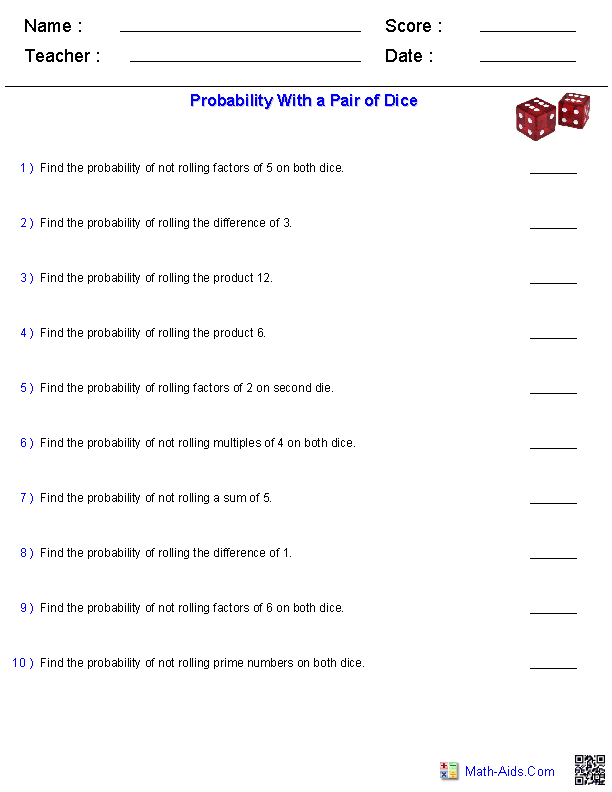##### Probability WorksheetsWith a Deck of Cards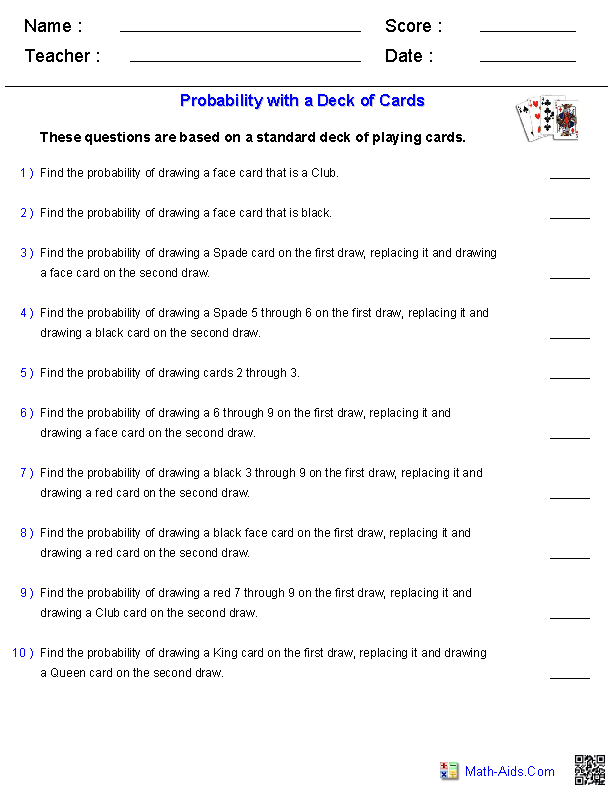##### Probability WorksheetsUsing a Spinner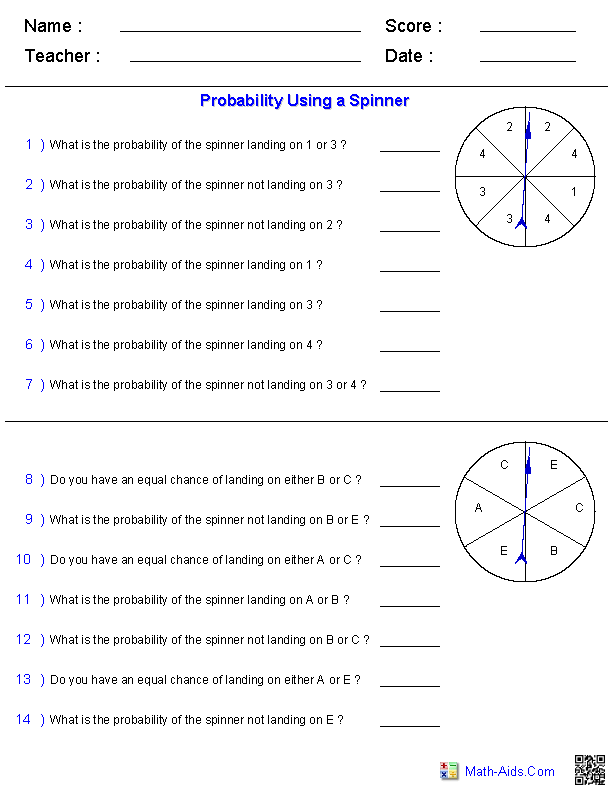Recommended Videos

## Detailed Description for All Probability Worksheets

Definitions of Probability Worksheet
These Probability Worksheets are a great handout for reinforcing the definitions of Probability.

Probability on Numbers Worksheet
These Probability Worksheets will produce problems with simple numbers between 1 and 50.

Probability With a Single Die Worksheet
These Probability Worksheets will produce problems with simple numbers, multiples, divisors, and factors using a single die.

Probability With a Pair of Dice Worksheet
These Probability Worksheets will produce problems with simple numbers, sums, differences, multiples, divisors, and factors using a pair of dice.

Probability With a Deck of Cards Worksheet
These Probability Worksheets will produce problems about a standard 52 card deck without the Jokers.

Probability Using a Spinner Worksheet
These Probability Worksheets will produce problems using a spinner.